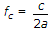# Electronics and Communication Engineering - Electromagnetic Field Theory - Discussion

10.

A rectangular metal waveguide filled with a dielectric of relative permittivity εr = 4, has the inside dimensions 3 x 1.2 cm, the cut off frequency for the dominant mode is

 [A]. 2.5 GHz [B]. 5 GHz [C]. 10 GHz [D]. 12.5 GHz

Answer: Option B

Explanation:for dominant mode.

 Kamlesh said: (Aug 18, 2014) Option A is correct. Phase velocity is c/2 hence f = c/4a.

 Vinay said: (Mar 10, 2015) Yes option A is correct. C' = C/2.

 Allaa said: (Sep 5, 2015) c = c0/sqrt(εr). f = c/2a.

 Ravi said: (May 21, 2016) Cut off frequency = c/(2a * sqrt (Er * Ur).

 Anjli said: (Jul 5, 2016) As f = C'/2a. And C' = C/2 (because Er = 4). So f = c/4a.

 Pk Rai said: (Aug 4, 2016) A is correct.

 Sunil Chavan said: (Jun 28, 2017) A is the answer.

 Ronston said: (Aug 10, 2017) What does 'a' stand for? dimension?

 Azar said: (Sep 20, 2017) fc = C/2a. fc = Cut-off Frequency. C = Speed of Light = 3 * 10^8, a = Width = 3 cm. fc = 3 * 10^8 / 2 *3 = 0.5 * 10^8 ==> 5 GHz.

 Vijay said: (Oct 20, 2017) Fc should be divided by 2.

 Parvez said: (Nov 1, 2017) Vp = c /sqrt(μrεr), here μr =1 ; εr = 4 (given). So, fc = Vp/2a. i.e. fc =c/(2a*sqrt(μrεr)). fc = 2.5 GHz.

 Ravi said: (Nov 10, 2017) 5 GHz is correct answer. fc = 1.5*10^8 sqrt{(m/a)^2+(n/b)^2} . Here m=1, n=0 (dominant mode in a rectangular waveguide is TE1,0). So,1.5*10^8 * m/a = 1.5*10^8 * 1/0.03 = 5*10^9 Hz. Here a is width.

 John Howard said: (Dec 30, 2017) Option A is correct. λc=2a; fc=v/λc or fc=(c/√(εr))/λc = 2.5 GHZ. v=1.5 10^8 m/s and λc=0.06 m.

 Priti said: (Apr 21, 2019) Where c is velocity of light. a =3, fc= 3 * 10^8/2 * 3 * 10^-3, = 5 * 10^9. = 5 GHz.

#### Post your comments here:

Name *:

Email   : (optional)

» Your comments will be displayed only after manual approval.

#### Current Affairs 2021

Interview Questions and Answers